Technical Article

# Determining Cut-Off Frequency and Transfer Function of RC High-Pass Filters

July 19, 2023 by Amna Ahmad

## Learn how to determine the RC high-pass filter's cut-off frequency and transfer function and plot gain/frequency and phase/frequency response graphs.

The RC high-pass filter is a circuit that allows high-frequency signals to pass through while attenuating low-frequency signals. It consists of a resistor (R) and a capacitor (C) connected in series. The filter operation is based on the time constant of the RC circuit, which determines the rate at which the capacitor charges and discharges.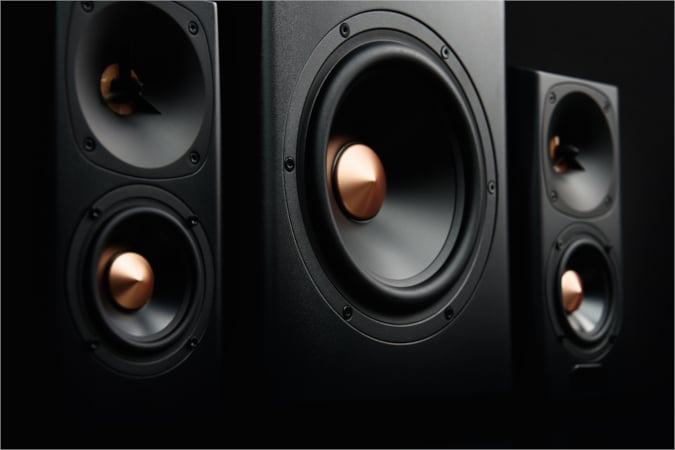##### Image used courtesy of Adobe Stock

The basic RC high-pass filter circuit in Figure 1(a) is seen to be similar to the RC low-pass circuit, except that the capacitor and resistor are interchanged. Here again, all input voltages are divided across R1 and XC1 [Figure 1(b)], and because the capacitive reactance is very high at low signal frequencies, low-frequency input voltages are heavily attenuated. As the signal frequency increases, XC1 becomes progressively smaller so high-frequency input voltages are only slightly attenuated.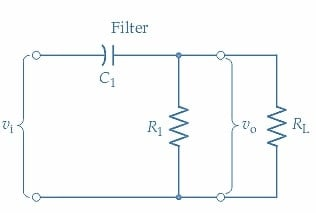##### (a) RC high-pass filter circuit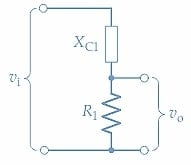### Frequency Response Graphs

A typical high-pass filter gain/frequency response graph is shown in Figure 2. It is seen that the output remains substantially equal to the input $$(v_{_o}/v_{_i}\approx0db)$$ for all frequencies above the cut-off frequency (fc). In this case, fc is the lower frequency at which the output voltage falls 3 dB from its normal (high frequency) level. For signal frequencies below fc, the output voltage decreases at 6 dB per octave. For the high-pass circuit, this is a 6 dB reduction each time the frequency is halved. The 6 dB per octave is equivalent to 20 dB per decade, meaning the filter gain is reduced by 20 dB whenever the signal frequency is reduced by 10. As in the case of the RC low-pass circuit, the cut-off frequency occurs when XC1=R1.

$f_{c}=\frac{1}{2\pi R_{1}C_{1}}$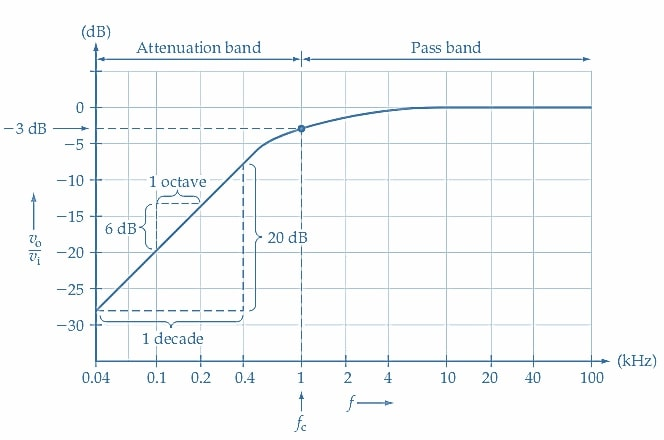##### Figure 2. Typical gain/frequency response graph for a high-pass filter. At the cut-off frequency (fc), the output level is 3 dB below the normal high-frequency output. At frequencies lower than fc, the gain falls off at 6 dB/octave or 20 dB/decade. Image used courtesy of Amna Ahmad

The high-pass filter phase/frequency response graph in Figure 3 shows that the output is substantially in phase with the input at high signal frequencies and increasingly leads the input as the signal frequency decreases. At the cut-off frequency, the phase lead is 45°, and below fc, the lead increases to a maximum of 90°. The resistor voltage leads the circuit input voltage by angle ɸ and that,

$\phi=\Big[\frac{X_{C1}}{R_{1}}\Big](1)$### Insertion Loss

Insertion loss in the passband of the high-pass filter results from the filter resistor effectively reducing the load resistance presented to the signal source. Refer to the circuit in Figure 1, and note that, without the circuit filter, the signal source's load is resistor RL. The signal voltage (vi) is divided across (source resistance) RS and RL to produce the output (vo). Normally, RL>>RS so that vo effectively equals vi. With the filter inserted, the capacitor offers a very low impedance in the circuit passband, and the load on the source becomes R1||RL. The input is now divided across RS and R1||RL, (see Example 1).

### High-Pass Filter Transfer Function

The transfer function, or input/output phasor relationship, for an RC high-pass filter, is easily derived from the voltage divider in Figure 1(b).

$\frac{v_{_o}}{v_{_i}}=\frac{R_{1}}{\sqrt{R^{2}_{1}+X^{2}_{C1}}}\angle tan^{-1}\Big(\frac{X_{C1}}{R_{1}}\Big)(2)$

Substituting $$R_{1}=\frac{1}{(2\pi f_{c}C_{1})}$$, and $$X_{C1}=\frac{1}{(2\pi fC_{1})}$$, Equation 2 becomes

$\frac{v_{_o}}{v_{_i}}=\Bigg(\frac{1}{\sqrt{\Big(\frac{f_{_c}}{f}}\Big)^{2}+1}\Bigg)\angle tan^{-1}\Big(\frac{f_{c}}{f}\Big)(3)$

Expressing $$\frac{v_{_o}}{v_{_i}}$$ in decibels

$\frac{v_{_o}}{v_{_i}}=20\,log\Bigg(\frac{1}{\sqrt{\Big(\frac{f_{_c}}{f}}\Big)^{2}+1}\Bigg)\angle tan^{-1}\Big(\frac{f_{c}}{f}\Big)(4)$

Equation 4 allows for rapid calculation of points on the gain and phase frequency response graphs at multiples of fc.

#### Example 1

Calculate the cut-off frequency for the high-pass filter circuit in Figure 4 and determine the effect of a 100 kΩ load resistance on the cut-off frequency. Also, determine the filter insertion loss if the signal source resistance is RS=100Ω.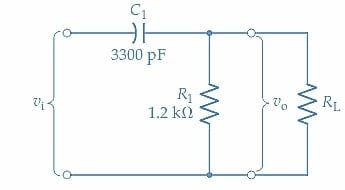#### Solution

Calculating the cut-off frequency

$f_{c}=\frac{1}{2\pi R_{1}C_{1}}=\frac{1}{2\pi\times1.2k\Omega\times3300pF}\approx40kHz$

For RL=100kΩ

$f_{c}=\frac{1}{2\pi (R_{1}||R_{L})C_{1}}=\frac{1}{2\pi\times(1.2k\Omega||100k\Omega)\times3300pF}\approx40.7kHz$

Without the filter in the circuit

For vi=1V

$v_{o1}=\frac{v_{_i}\times R_{L}}{R_{S}+R_{L}}=\frac{1V\times100k\Omega}{100\Omega+100k\Omega}\approx1V$

With the filter in the circuit, and the signal frequency in the pass band,

For vi=1V

$v_{o2}=\frac{v_{_i}\times (R_{1}||R_{L})}{R_{S}+(R_{1}||R_{L})}=\frac{1V\times(1.2k\Omega||100k\Omega)}{100\Omega+(1.2k\Omega||100k\Omega)}\approx0.9V$

Filter Insertion Loss

$Insertion\,Loss=20\,log\,log\Big(\frac{v_{o2}}{v_{o1}}\Big)=20\,log\Big(\frac{0.9V}{1V}\Big)\approx-0.9dB$

### RC High-Pass Filter Circuit Takeaways

The RC high-pass filter circuit consists of a resistor and a capacitor, which work together to attenuate low-frequency signals while allowing high-frequency signals to pass through. The cut-off frequency of the filter can be determined by the values of the resistor and capacitor. The transfer function describes the relationship between the input and output signals of the filter, and the gain/frequency and phase/frequency response graphs provide a visual representation of how the filter affects different frequencies.